# COMBINA function

In Excel we have lots of functions, in which COMBINA function is one of the Math & trig function. This function has been introduced with Excel 2013.  It returns the number of combinations, with repetitions, for a given number of objects.

Syntax of COMBINA function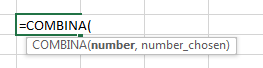Arguments:

Number is the total number of objects available.

Number_chosen is the number of combinations you require.

Let’s take an example and understand how to use COMBINA function:-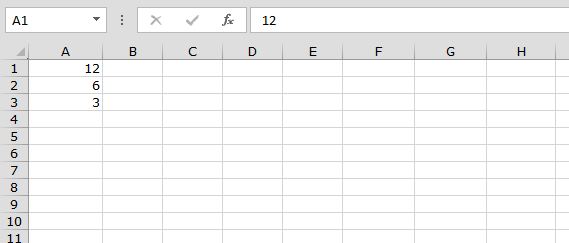• Enter the formula in cell C1
• =COMBINA(A1,A2)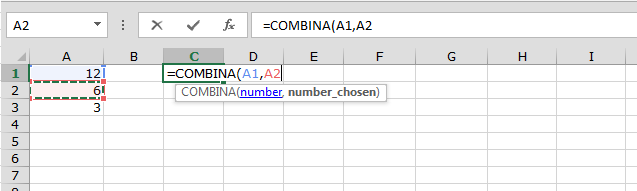• Press Enter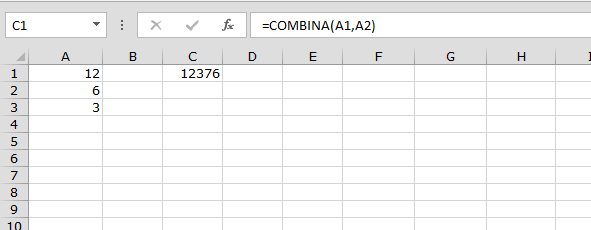• The function will return 12376, it means we can create 12376 combinations

Now we will change the chosen number:-

• Enter formula in cell C2
• =COMBINA(A1,A3)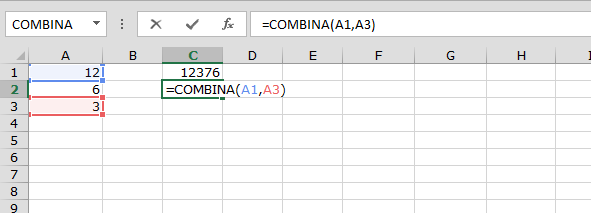• Press Enter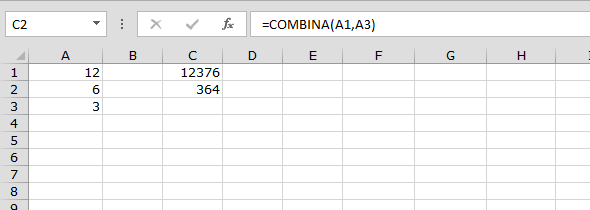• We can make 364 sets

This is how we can use COMBINA function in Microsoft Excel.

In the above image we can see that syntax is showing the range name, sum of the months number are also correct. In this way we use range name as syntax in Microsoft Excel.We would love to hear from you, do let us know how we can improve, complement or innovate our work and make it better for you. Write us at info@exceltip.com

1.I only recently started playing with the new functions in this version and was confused about the output of the function relative to the description by Microsoft. Thanks for explaining it.

However, the criteria are not quite right as COMBINA(0,0) is the only non-error output when N=0 yet this should be equal to COMBIN(-1,-1) which returns an error. I'm not sure why it provides such a result.

In fact the criteria provided by Microsoft is wrong as it states that Number > NumberChosen.

Presuming that COMBINA(N,M) = COMBIN(N+M-1,N-1)

N-1>=0 --> N>0
N+M-1>=N-1 --> M>=0

The only exception is when N=M=0 as noted earlier.

Terms and Conditions of use

The applications/code on this site are distributed as is and without warranties or liability. In no event shall the owner of the copyrights, or the authors of the applications/code be liable for any loss of profit, any problems or any damage resulting from the use or evaluation of the applications/code.